We think you are located in United States. Is this correct?

We notice that the gradient of a curve changes at every point on the curve, therefore we need to work with the average gradient. The average gradient between any two points on a curve is the gradient of the straight line passing through the two points.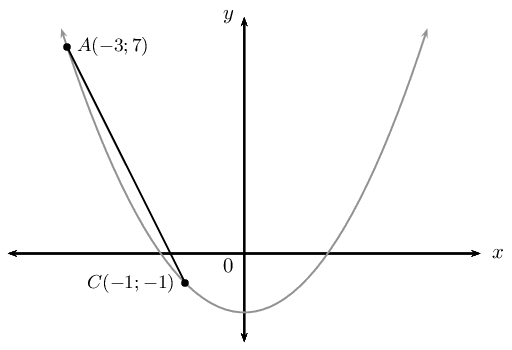For the diagram above, the gradient of the line $$AC$$ is \begin{align*} \text{Gradient }&= \frac{y_A - y_C}{x_A - x_C} \\ &= \frac{7-(-1)}{-3-(-1)} \\ &= \frac{8}{-2} \\ &= -4 \end{align*} This is the average gradient of the curve between the points $$A$$ and $$C$$.

What happens to the gradient if we fix the position of one point and move the second point closer to the fixed point?

## Gradient at a single point on a curve

The curve shown here is defined by $$y=-2{x}^{2}-5$$. Point $$B$$ is fixed at $$\left(0;-5\right)$$ and the position of point $$A$$ varies.

Complete the table below by calculating the $$y$$-coordinates of point $$A$$ for the given $$x$$-coordinates and then calculating the average gradient between points $$A$$ and $$B$$.$$x_A$$ $$y_A$$ Average gradient $$-\text{2}$$ $$-\text{1,5}$$ $$-\text{1}$$ $$-\text{0,5}$$ $$\text{0}$$ $$\text{0,5}$$ $$\text{1}$$ $$\text{1,5}$$ $$\text{2}$$
1. What happens to the average gradient as $$A$$ moves towards $$B$$?
2. What happens to the average gradient as $$A$$ moves away from $$B$$?
3. What is the average gradient when $$A$$ overlaps with $$B$$?

In the example above, the gradient of the straight line that passes through points $$A$$ and $$C$$ changes as $$A$$ moves closer to $$C$$. At the point where $$A$$ and $$C$$ overlap, the straight line only passes through one point on the curve. This line is known as a tangent to the curve.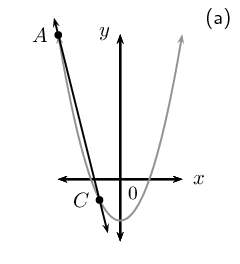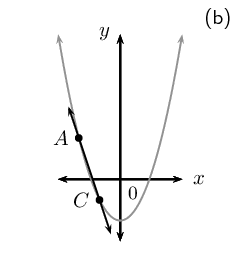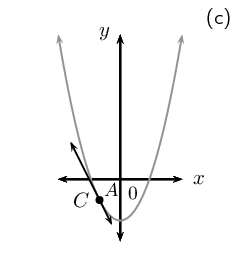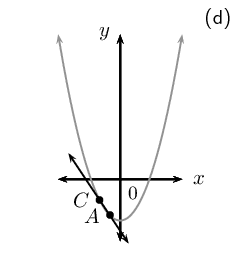We therefore introduce the idea of the gradient at a single point on a curve. The gradient at a point on a curve is the gradient of the tangent to the curve at the given point.

## Worked example 7: Average gradient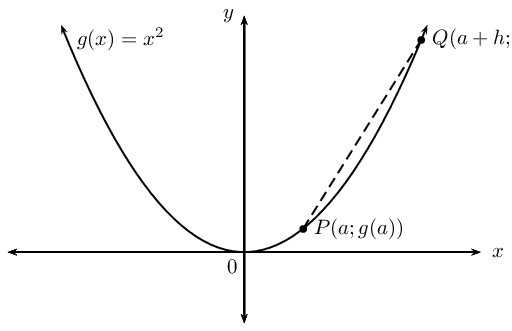1. Find the average gradient between two points $$P\left(a;g(a)\right)$$ and $$Q\left(a+h;g(a+h)\right)$$ on a curve $$g(x)={x}^{2}$$.
2. Determine the average gradient between $$P\left(2;g(2)\right)$$ and $$Q\left(5;g(5)\right)$$.
3. Explain what happens to the average gradient if $$Q$$ moves closer to $$P$$.

### Assign labels to the $$x$$-values for the given points

\begin{align*} x_1 &= a \\ x_2 &= a + h \end{align*}

### Determine the corresponding $$y$$-coordinates

Using the function $$g(x) = x^2$$, we can determine: $y_1 = g(a) = a^2$ \begin{align*} y_2 &= g(a + h) \\ &= (a + h)^2 \\ &= a^2 + 2ah + h^2 \end{align*}

\begin{align*} \frac{{y}_{2}-{y}_{1}}{{x}_{2}-{x}_{1}} &= \frac{\left({a}^{2}+2ah+{h}^{2}\right)-\left({a}^{2}\right)}{\left(a+h\right)-\left(a\right)} \\ &= \frac{{a}^{2}+2ah+{h}^{2}-{a}^{2}}{a+h-a} \\ &= \frac{2ah+{h}^{2}}{h} \\ &= \frac{h(2a+h)}{h} \\ &= 2a+h \end{align*} The average gradient between $$P\left(a;g(a)\right)$$ and $$Q\left(a+h;g(a+h)\right)$$ on the curve $$g(x)={x}^{2}$$ is $$2a+h$$.

### Calculate the average gradient between $$P\left(2;g(2)\right)$$ and $$Q\left(5;g(5)\right)$$

The $$x$$-coordinate of $$P$$ is $$a$$ and the $$x$$-coordinate of $$Q$$ is $$a+h$$ therefore if we know that $$a=2$$ and $$a+h=5$$, then $$h=3$$.

$$2a+h=2\left(2\right)+\left(3\right)=7$$

### When $$Q$$ moves closer to $$P$$

When point $$Q$$ moves closer to point $$P$$, $$h$$ gets smaller.

When the point $$Q$$ overlaps with the point $$P$$, $$h=0$$ and the gradient is given by $$2a$$.

We can write the equation for average gradient in another form. Given a curve $$f(x)$$ with two points $$P$$ and $$Q$$ with $$P\left(a;f(a)\right)$$ and $$Q\left(a+h;f(a+h)\right)$$. The average gradient between $$P$$ and $$Q$$ is: \begin{align*} \text{Average gradient }&= \frac{{y}_{Q}-{y}_{P}}{{x}_{Q}-{x}_{P}} \\ &= \frac{f(a+h)-f(a)}{\left(a+h\right)-\left(a\right)} \\ &= \frac{f(a+h)-f(a)}{h} \end{align*} This result is important for calculating the gradient at a point on a curve and will be explored in greater detail in Grade $$\text{12}$$.

## Worked example 8: Average gradient

Given $$f(x) = -2x^2$$.

1. Draw a sketch of the function and determine the average gradient between the points $$A$$, where $$x = 1$$, and $$B$$, where $$x = 3$$.

2. Determine the gradient of the curve at point $$A$$.

### Examine the form of the equation

From the equation we see that $$a < 0$$, therefore the graph is a “frown” and has a maximum turning point. We also see that when $$x = 0$$, $$y = 0$$, therefore the graph passes through the origin.

### Draw a rough sketch### Calculate the average gradient between $$A$$ and $$B$$

\begin{align*} \text{Average gradient} &= \frac{f(3) - f(1)}{3 - 1} \\ &= \frac{-2(3)^2 - (-2(1)^2)}{2} \\ &= \frac{-18 + 2}{2} \\ &= \frac{-16}{2} \\ &= -8 \end{align*}

### Calculate the average gradient for $$f(x)$$

\begin{align*} \text{Average gradient} &= \frac{f(a + h) - f(a)}{(a + h) - a} \\ &= \frac{-2(a + h)^2 - (-2a^2)}{h} \\ &= \frac{-2a^2 - 4ah - 2h^2 + 2a^2}{h} \\ &= \frac{-4ah - 2h^2}{h} \\ &= \frac{h(-4a - 2h)}{h} \\ &= -4a - 2h \end{align*} At point $$A$$, $$h = 0$$ and $$a = 1$$.

Therefore \begin{align*} \text{Average gradient} &= -4a - 2h \\ &= -4(1) - 2(0) \\ &= -4 \end{align*}

Textbook Exercise 5.8

Determine the average gradient of the curve $$f(x)=x\left(x+3\right)$$ between $$x=5$$ and $$x=3$$.

$$\text{11}$$

Hence, state what you can deduce about the function $$f$$ between $$x=5$$ and $$x=3$$.

Solution not available at present

$$A\left(1;3\right)$$ is a point on $$f(x)=3{x}^{2}$$.

Draw a sketch of $$f(x)$$ and label point $$A$$.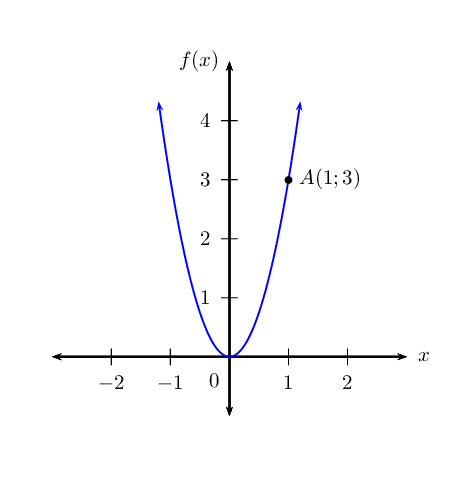Determine the gradient of the curve at point $$A$$.

$$\text{6}$$

Determine the equation of the tangent line at $$A$$.

$$y = 6x - 3$$

Given: $$g(x) = -x^2 + 1$$.

Draw a sketch of $$g(x)$$.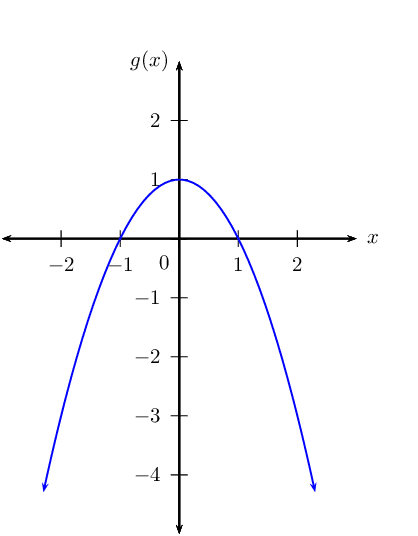Determine the average gradient of the curve between $$x=-2$$ and $$x=1$$.

$$\text{1}$$

Determine the gradient of $$g$$ at $$x=2$$.

$$\text{4}$$

Determine the gradient of $$g$$ at $$x=0$$.

$$\text{0}$$